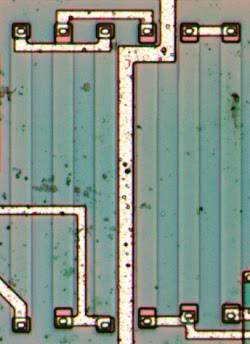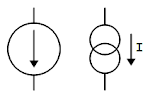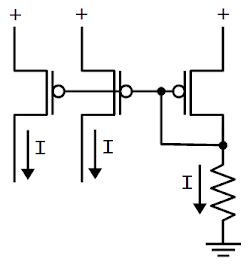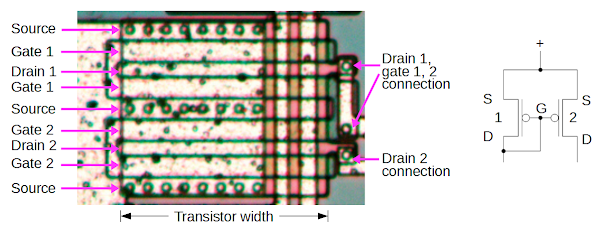Browse over 10,000 Electronics Projects

# Reverse engineering the popular 555 timer chip (CMOS version)### How resistors are implemented in silicon

Resistors are a key component of analog circuits. Unfortunately, resistors in ICs are large and inaccurate; the resistances can vary by 50% from chip to chip. Thus, analog ICs are designed so only the ratio of resistors matters, not the absolute values, since the ratios remain nearly constant even if the values vary depending on manufacturing conditions.These resistors form the voltage divider in the CMOS 555 timer.

The photo above shows the resistors that form the voltage divider in the chip.
There are six 50kΩ resistors, connected in series to form three 100kΩ resistors.
The resistors are the pale vertical rectangles.
At the end of each resistor, a via and P+ silicon well (pink square) connects the resistor to the metal layer, which wires them together. The resistors themselves are probably P-doped silicon.

To reduce current, the CMOS chip uses 100kΩ resistors, much larger than the 5kΩ resistors in the bipolar 555 timer.
Urban legend says that the 555 is named after these three 5K resistors, but
according to its designer
555 is just an arbitrary number in the 500 chip series

### IC component: The current mirror

There are some subcircuits that are very common in analog ICs, but may seem mysterious at first. The current mirror is one of these.
If you’ve looked at analog IC block diagrams, you may have seen the symbols below, indicating a current source, and wondered what a current source is and why you’d use one.Schematic symbols for a current source.

The idea of the current mirror is you start with one known current and then you can “clone” multiple copies of the current with a simple transistor circuit, the current mirror.
A common use of a current mirror is to replace resistors. As explained earlier, resistors inside ICs are both inconveniently large and inaccurate. It saves space to use a current mirror instead of a resistor whenever possible.
Also, the currents produced by a current mirror are nearly identical, unlike the currents produced by two resistors.

The circuit below shows how a current mirror is implemented with three identical transistors. A reference current passes through the transistor on the right. (In this case, the current is set by the resistor.) Since all the transistors have the same emitter voltage and base voltage, they source the same current, so the currents on the left match the reference current on the right.
For more flexibility, you can modify the relative sizes of the transistors in the current mirror and make the copied current larger or smaller than the reference current. The CMOS 555 chip uses a variety of transistor sizes to control the currents in the circuit.A current mirror formed from PMOS transistors. The left two currents mirror the current on the right, which is controlled by the resistor.

The diagram below shows one of the current mirrors in the LMC555 chip, formed from two transistors.
Each transistor is actually two transistors in parallel, which is a common trick in the chip, so there are physically two pairs of transistors.
It’s a bit tricky to see the transistors because the metal layer partially covers them, but hopefully the description will make sense.
Starting at the top, the first transistor is formed from the wide rectangles for source, gate 1, and drain 1. Note the vias connecting the metal layer to the source.
The next transistor shares drain 1, with the second gate 1 and source below.
Since these two transistors share the drain, and the sources and gates are wired the same, the two transistors effectively form one larger transistor.
Likewise, there are two transistors below in parallel: source, gate 2, drain 2, and then drain2, gate2, source.Two pairs of PMOS transistors in the LMC555 chip form a current mirror.

The schematic on the right shows how the transistors are wired together as a current mirror.
If you look at the photo carefully, you can see that a single polysilicon strip snakes back and forth to form all the gates, so the gates are connected together.
On the right, the upper metal strip connects drain 1 and the gates to the rest of the circuit.
The lower metal strip is connected to drain 2.

Pages: 1 2 3 4 5 6

Top